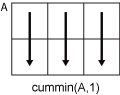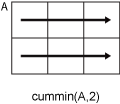# cummin

Cumulative minimum

## Syntax

``M = cummin(A)``
``M = cummin(A,dim)``
``M = cummin(___,direction)``
``M = cummin(___,nanflag)``

## Description

example

````M = cummin(A)` returns the cumulative minimum elements of `A`.If `A` is a vector, then `M` is a vector of the same size and type and contains the cumulative minima of `A`.If `A` is a matrix, then `M` is a matrix of the same size and type and contains the cumulative minima in each column of `A`.If `A` is a multidimensional array, then `M` is an array of the same size and type and contains the cumulative minima along the first array dimension of `A` whose size is greater than 1.If `A` is a table or timetable, then `cummin(A)` returns a table or timetable containing the cumulative minima of each variable. (since R2023a)```

example

````M = cummin(A,dim)` returns the cumulative minima along dimension `dim`. For example, if `A` is a matrix, then `cummin(A,2)` returns the cumulative minima along the rows of `A`.```

example

````M = cummin(___,direction)` specifies the direction for any of the previous syntaxes. For example, `cummin(A,2,"reverse")` returns the cumulative minima of `A` by working from end to beginning of the second dimension of `A`.```

example

````M = cummin(___,nanflag)` specifies whether to omit or include `NaN` values in `A`. For example, `cummin(A,"includenan")` includes `NaN` values when computing each minimum. By default, `cummin` omits `NaN` values.```

## Examples

collapse all

Find the cumulative minima of a 1-by-10 vector of random integers.

`v = randi([0,10],1,10)`
```v = 1×10 8 9 1 10 6 1 3 6 10 10 ```
`M = cummin(v)`
```M = 1×10 8 8 1 1 1 1 1 1 1 1 ```

Find the cumulative minima of the columns of a 3-by-3 matrix.

`A = [3 5 2; 1 6 3; 7 8 1]`
```A = 3×3 3 5 2 1 6 3 7 8 1 ```
`M = cummin(A)`
```M = 3×3 3 5 2 1 5 2 1 5 1 ```

Find the cumulative minima of the rows of a 3-by-3 matrix.

`A = [3 5 2; 1 6 3; 7 8 1]`
```A = 3×3 3 5 2 1 6 3 7 8 1 ```
`M = cummin(A,2)`
```M = 3×3 3 3 2 1 1 1 7 7 1 ```

Calculate the cumulative minima in the third dimension of a 2-by-2-by-3 array. Specify `direction` as `"reverse"` to work from the end of the third dimension to the beginning.

`A = cat(3,[1 2; 3 4],[9 10; 11 12],[5 6; 7 8])`
```A = A(:,:,1) = 1 2 3 4 A(:,:,2) = 9 10 11 12 A(:,:,3) = 5 6 7 8 ```
`M = cummin(A,3,"reverse")`
```M = M(:,:,1) = 1 2 3 4 M(:,:,2) = 5 6 7 8 M(:,:,3) = 5 6 7 8 ```

Create a matrix containing `NaN` values.

`A = [3 5 NaN 4; 2 6 2 9; 1 3 0 NaN]`
```A = 3×4 3 5 NaN 4 2 6 2 9 1 3 0 NaN ```

Compute the cumulative minima of the matrix, including `NaN` values. For matrix columns that contain any `NaN` value, the cumulative minimum values are `NaN` as soon as the first `NaN` value is encountered.

`M = cummin(A,"includenan")`
```M = 3×4 3 5 NaN 4 2 5 NaN 4 1 3 NaN NaN ```

## Input Arguments

collapse all

Input array, specified as a vector, matrix, multidimensional array, table, or timetable. For complex elements, `cummin` compares the magnitude of the elements. If magnitudes are equal, `cummin` also compares the phase angles.

Data Types: `single` | `double` | `int8` | `int16` | `int32` | `int64` | `uint8` | `uint16` | `uint32` | `uint64` | `logical` | `duration` | `table` | `timetable`
Complex Number Support: Yes

Dimension to operate along, specified as a positive integer scalar. If you do not specify the dimension, then the default is the first array dimension of size greater than 1.

Consider a two-dimensional input array, `A`:

• `cummin(A,1)` works on successive elements in the columns of `A` and returns an array of the same size as `A` with the cumulative minima in each column.• `cummin(A,2)` works on successive elements in the rows of `A` and returns an array of the same size as `A` with the cumulative minima in each row.`cummin` returns `A` if `dim` is greater than `ndims(A)`.

Direction of cumulation, specified as one of these values:

• `"forward"` — Work from `1` to `end` of the operating dimension.

• `"reverse"` — Work from `end` to `1` of the operating dimension.

Missing value condition, specified as one of these values:

• `"omitmissing"` or `"omitnan"` — Ignore `NaN` values in `A` when computing the cumulative minima. If `A` has consecutive leading `NaN` values, then the corresponding elements in `M` are `NaN`. `"omitmissing"` and `"omitnan"` have the same behavior.

• `"includemissing"` or `"includenan"` — Include `NaN` values in `A` when computing the cumulative minima. Elements in `M` are `NaN` as soon as the first `NaN` value in `A` is encountered. `"includemissing"` and `"includenan"` have the same behavior.

## Tips

• The `"reverse"` option in many cumulative functions allows quick directional calculations without requiring a flip or reflection of the input array.

## Version History

Introduced in R2014b

expand all